# GRE Math : How to find the perimeter of an acute / obtuse isosceles triangle

## Example Questions

2 Next →

### Example Question #11 : Triangles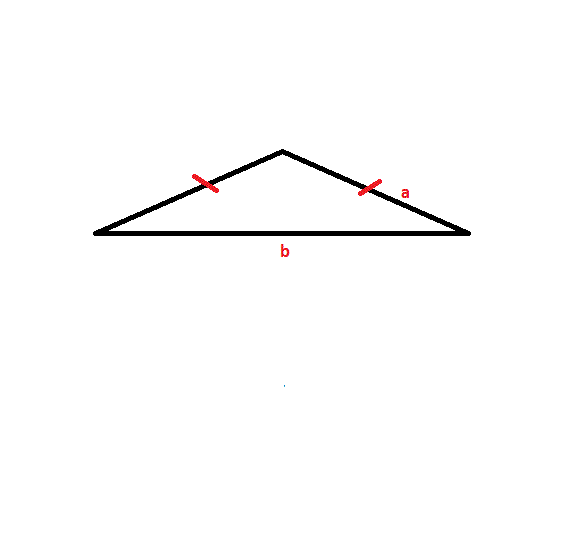The obtuse Isosceles triangle shown above has two sides with lengthand one side length. The length of side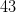inches. Side length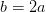. Find the perimeter of the triangle.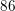inches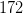inches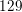inches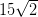inches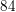inchesinches

Explanation:

To find the perimeter of this triangle, apply the perimeter formula: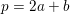Since,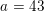, andthenmust have a value of: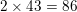This triangle has two side lengths ofinches, and one side length ofinches.

Thus, the solution is: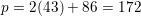### Example Question #12 : Isosceles Triangles

A triangle has two sides with lengthand one side length. The length of side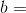yard. If the length of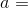the length of side, what is the perimeter of the triangle?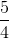yard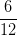yard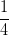yard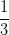yard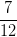yardyard

Explanation:

The first step to solving this problem is that we must find the length of lengthSince,is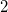4 the length of side, use the following steps: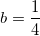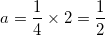Now, apply the formula: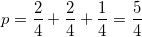2 Next →

Tired of practice problems?

Try live online GRE prep today.